# Python Function Tutorial

## 1- Python Function

Function is a special command block, it is named and it helps program code to be more easily to read, and it is called to use in different places in the program. The function is a reuseable command block.

### Syntax:

** function syntax **
```def functionName( parameters ):
"Short description of the function"
codes ...
return [expression]
```
• A function starts with word "def" (define) and followed by the function's name.
• Next, it is the list of parameters in the brackets () and colon (:), the function can include 0, 1 or parameters, the parameters are separated by comma.
• The first line of the function body is a short description of the function (optional).

### return statement:

The return statement is used to returns a value (or an expression), or "nothing". When the return statement is run, function will end. Return is a statement not required in the function body.
 Example Description return 3 Function return a value, and ends return Function return nothing, and ends

### Parameters:

Function includes 0, 1 or parameters separated by comma. There are four types of parameters:
1. Required Parameter
2. Default parameter
3. Variable-Length Parameter
4. Keyword Parameter

## 2- Function examples

For example, a function has a parameter, and returns "Nothing".
functionExample1.py
```# Define a function:
def sayHello(name)  :

# If name is empty or null.
if not name   :
print( "Hello every body!" )

# If name is not empty and not null.
else :
print( "Hello " + name)

# Call function, pass parameters to function.
sayHello("")

sayHello("Python");

sayHello("Java");
```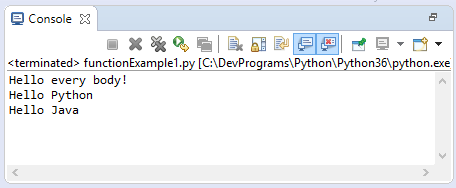Next is the example of a function that has a parameter and returns the value.
functionExample2.py
```# Define a function:
def getGreeting(name)  :

# If name is empty or null (None).
if not name   :
# Returns a value.
# And function ends here.
return "Hello every body!"

# If name is not empty and not null (not None),
# this code will be executed.
return "Hello " + name

# Call function, pass parameters to function.
greeting = getGreeting("")

print(greeting)

greeting = getGreeting("Python")

print(greeting)
```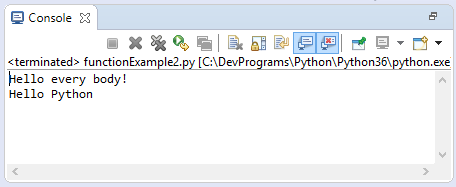## 3- Function with required parameters

The following example defines the showInfo function having 2 parameters. Both of the parameters are required. When you call for the function, you need to provide 2 parameters to the function. Conversely program will throw an error.
requiredParameterExample.py
```
def showInfo(name, gender):

print ("Name: ", name);
print ("Gender: ", gender);

# Valid
showInfo("Tran", "Male")

# Invalid ==> Error!!
showInfo("Tran")
```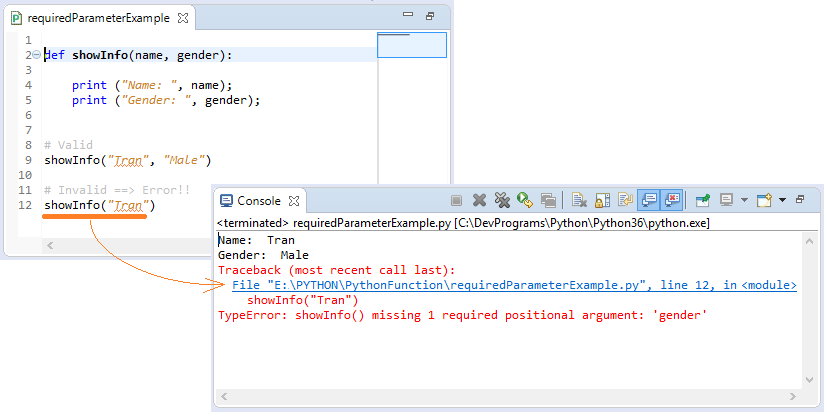## 4- Function with default parameters

Function can have a lot of  parameters, including the required parameters and ones with default values.
The showInfo function below has three parameters (name, gender = "Male", country = "US"):
1. name is a required parameter.
2. gender is the parameter with default value "Male".
3. country is a parameter with the default value "US".
defaultParameterExample.py
```
def showInfo(name, gender = "Male", country ="US"):

print ("Name: ", name)
print ("Gender: ", gender)
print ("Country: ", country)

# Valid

print (" ------ ")

# Valid
showInfo("Tom", "Male")

print (" ------ ")

# Valid
showInfo("Jerry")

print (" ------ ")

# Valid
showInfo(name = "Tintin", country ="France")

print (" ------ ")

```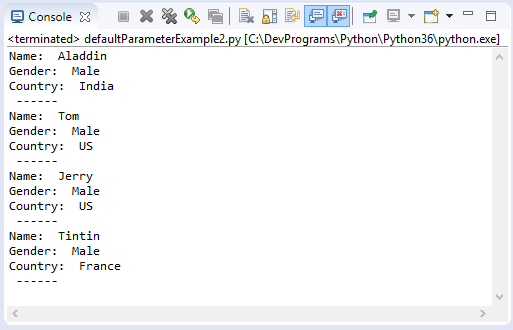## 5- Function with Variable-Length parameter

Variable-length Parameter is a special parameter. When calling for function, you can pass 0, 1 or values to that parameter.

Note: "Variable-length Parameter" must always be the last parameter of the function.

### Example:

sumValues function has three parameters:
• Parameters a, b are required.
• Parameters *others are "Variable-Length Parameters".
variableLengthParameterExample.py
```def sumValues(a, b, *others):

retValue = a + b

# 'others' parameter like an array.
for other in others :
retValue = retValue + other

return retValue
```
How to call for functions:
testVariableLengthParameter.py
```from variableLengthParameterExample import sumValues

# Pass: *others = []
a = sumValues(10, 20)

print("sumValues(10, 20) = ", a);

# Pass: *others = 
a = sumValues(10, 20, 1);

print("sumValues(10, 20, 1 ) = ", a);

# Pass: *others = [1,2]
a = sumValues(10, 20, 1, 2);

print("sumValues(10, 20, 1 , 2) = ", a);

# Pass: *others = [1,2,3,4,5]
a = sumValues(10, 20, 1, 2,3,4,5);

print("sumValues(10, 20, 1, 2, 3, 4, 5) = ", a);
```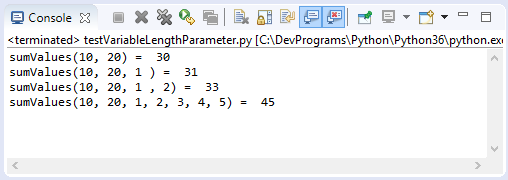## 6- Anonymous function

Functions are called anonymous if they are not defined in the usual way by the def keyword, which uses the lambda keyword.
1. Anonymous functions can have 0 or more parameters, but only one expression in the body of the function. The value of the expression is the return value of the function. But do not use the keyword 'return'.
2. List of parameters separated by commas, and not enclosed in parenthesis ( ).
3. In the anonymous function's body, you can not access external variables, you can only access its parameters.
4. An anonymous function cannot be a direct call to print because lambda requires an expression

### Syntax:

* syntax *
```
lambda [arg1 [,arg2,.....argn]]  :  expression
```
Example:
lambdaFunctionExample.py
```# Declare a variable: hello = an anonymous function have no parameter.
hello = lambda  : "Hello"

# Declare a variable: mySum = an anonymous function with 2 parameters.
mySum = lambda a, b : a + b

a= hello()

print (a)

a = mySum(10, 20)

print (a)
```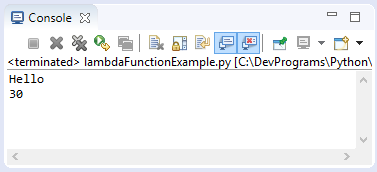#### View more Tutorials:

Maybe you are interested

These are online courses outside the o7planning website that we introduced, which may include free or discounted courses.

•New to Python Automation..?Try Step by Step Python 4 Testers
•Learn Programming in Python With the Power of Animation
•Python Programming Full Course (Basics,OOP,Modules,PyQt)
•Python Fundamentals
•Python GUI : From A-to-Z With 2 Final Projects
•Python MySql From Scratch
•Master Computer Vision™ OpenCV4 in Python with Deep Learning
•Python BeautifulSoup: Extract Web Data Beautifully
•Python 3000: Tactical File I/O
•Learning Path: Python: Design and Architect Python Apps
•Learn iPython: The Full Python IDE
•Learning Path: Python GUI Projects
•Python 3 For Beginner - Object-Oriented Programming
•Python 2000: Beyond The Basics
•Learning iPython Notebook